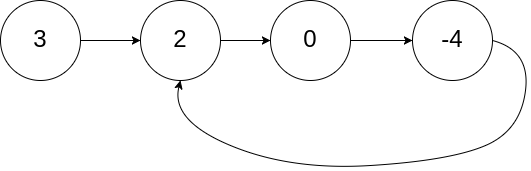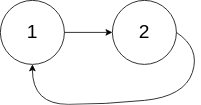# 141. Linked List Cycle (Easy)

Given a linked list, determine if it has a cycle in it.

To represent a cycle in the given linked list, we use an integer `pos` which represents the position (0-indexed) in the linked list where tail connects to. If `pos` is `-1`, then there is no cycle in the linked list.

Example 1:

```Input: head = [3,2,0,-4], pos = 1
Output: true
Explanation: There is a cycle in the linked list, where tail connects to the second node.
```Example 2:

```Input: head = [1,2], pos = 0
Output: true
Explanation: There is a cycle in the linked list, where tail connects to the first node.
```Example 3:

```Input: head = , pos = -1
Output: false
Explanation: There is no cycle in the linked list.
```Can you solve it using O(1) (i.e. constant) memory?

## Solutions

### 1.

``````class Solution {

// Merge the followup element until the ring remains only one element
// which is the entry of the ring.

return true;
}

}

return false;
}
}
``````

### 2.

``````class Solution {
// use a set to keep all the visited elements
Set<ListNode> visited = new HashSet<>();

while (node != null) {
if (visited.contains(node)) {
return true;
}

node = node.next;
}

return false;
}
}
``````